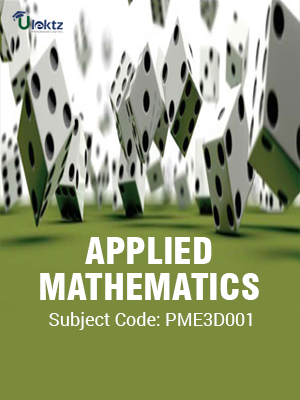•My WalletMy Order
•My Profile
•My Connections
•My Books
•My Videos
•My Tests
•My Calender
•My Messages
•My Shopping Cart
•My Orders
•Account Settings
•Help

# Book Details# APPLIED MATHEMATICS

 Course Code : PME3D001 Author : uLektz University : Biju Patnaik University of Technology (BPUT) Regulation : 2016 Categories : Mechanical Format :ePUB3 (DRM Protected) Type : eBook

FREE

Description :APPLIED MATHEMATICS of PME3D001 covers the latest syllabus prescribed by Biju Patnaik University of Technology (BPUT) for regulation 2016. Author: uLektz, Published by uLektz Learning Solutions Private Limited.

Note : No printed book. Only ebook. Access eBook using uLektz apps for Android, iOS and Windows Desktop PC.

##### Topics
###### UNIT - I PROBABILITY AND STATISTICS

1.1 Probability, Random variables, Probability distributions

1.2 Mean and variance of distribution, Binomial, Poisson and Hyper geometric distributions

1.3 Normal and exponential distribution, Distribution of several random variables

1.4 Statistics: Random sampling, Estimation of Parameters, Confidence Intervals

1.5 Testing of hypothesis, Acceptance sampling

1.6 Regression Analysis, Fitting Straight Lines, Correlation analysis

###### UNIT - II PARTIAL DIFFERENTIAL EQUATION

2.1 Partial differential equation of first order, Linear partial differential equation, Non-linear partial differential equation

2.2 Homogenous and non-homogeneous partial differential equation with constant co-efficient, Cauchy type, Monge’s method

2.3 Second order partial differential equation

2.4 The vibrating string, the wave equation and its solution, the heat equation and its solution, Two dimensional wave equation and its solution

2.5 Laplace equation in polar, cylindrical and spherical coordinates

###### UNIT - III COMPLEX ANALYSIS AND COMPLEX INTEGRATION

3.1 Complex Analysis: Analytic function, Cauchy-Riemann equations

3.2 Laplace equation, Conformal mapping

3.3 Complex integration: Line integral in the complex plane, Cauchy’s integral theorem, Cauchy’s integral formula, Derivatives of analytic functions

###### UNIT - IV POWER SERIES

4.1 Power Series, Taylor’s series, Laurent’s series

4.2 Singularities and zeros

4.3 Residue integration method, Evaluation of real integrals

### Related Books